# The NLMIXED Procedure

### Restricting the Step Length

Almost all line-search algorithms use iterative extrapolation techniques that can easily lead them to (feasible) points where the objective function f is no longer defined or is difficult to compute. Therefore, PROC NLMIXED provides options restricting the step lengthor trust region radius
, especially during the first main iterations.

The inner product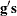of the gradient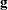and the search directionis the slope of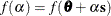along the search direction. The default starting value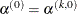in each line-search algorithm (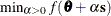) during the main iteration k is computed in three steps:

1. The first step uses either the difference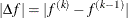of the function values during the last two consecutive iterations or the final step-size value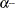of the last iteration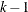to compute a first value of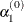.

• If the DAMPSTEP option is not used,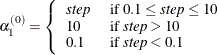with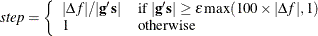This value ofcan be too large and can lead to a difficult or impossible function evaluation, especially for highly nonlinear functions such as the EXP function.

• If the DAMPSTEP= r option is used,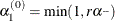The initial value for the new step length can be no larger than r times the final step lengthof the former iteration. The default value is r = 2.

2. During the first five iterations, the second step enables you to reduceto a smaller starting value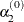by using the INSTEP= r option: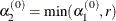After more than five iterations,is set to.

3. The third step can further reduce the step length by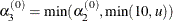where u is the maximum length of a step inside the feasible region.

The INSTEP= r option enables you to specify a smaller or larger radiusof the trust region used in the first iteration of the trust region and double-dogleg algorithms. The default initial trust region radius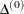is the length of the scaled gradient (Moré, 1978). This step corresponds to the default radius factor of r = 1. In most practical applications of the TRUREG and DBLDOG algorithms, this choice is successful. However, for bad initial values and highly nonlinear objective functions (such as the EXP function), the default start radius can result in arithmetic overflows. If this happens, you can try decreasing values of INSTEP= r, 0 < r < 1, until the iteration starts successfully. A small factor r also affects the trust region radius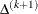of the next steps because the radius is changed in each iteration by a factor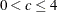, depending on the ratio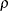expressing the goodness of quadratic function approximation. Reducing the radiuscorresponds to increasing the ridge parameter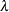, producing smaller steps aimed more closely toward the (negative) gradient direction.